Next: Variational Methods Up: Time-Dependent Perturbation Theory Previous: Intensity Rules

# Forbidden Transitions

Atomic transitions which are forbidden by the electric dipole selection rules (1149) and (1150) are unsurprisingly known as forbidden transitions. It is clear from the analysis in Sect. 13.8 that a forbidden transition is one for which the matrix element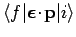is zero. However, this matrix element is only an approximation to the true matrix element for radiative transitions, which takes the form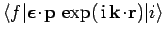. Expanding, and keeping the first two terms, the matrix element for a forbidden transition becomes(1164)

Hence, if the residual matrix element on the right-hand side of the above expression is non-zero then a forbidden'' transition can take place, allbeit at a much reduced rate. In fact, in Sect. 13.9, we calculated that the typical rate of an electric dipole transition is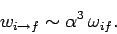(1165)

Since the transition rate is proportional to the square of the radiative matrix element, it is clear that the transition rate for a forbidden transition enabled by the residual matrix element (1164) is smaller than that of an electric dipole transition by a factor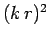. Estimatingas the Bohr radius, andas the wavenumber of a typical spectral line of hydrogen, it is easily demonstrated that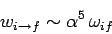(1166)

for such a transition. Of course, there are some transitions (in particular, thetransition) for which the true radiative matrix elementis zero. Such transitions are absolutely forbidden.

Finally, it is fairly obvious that excited states which decay via forbidden transitions have much longer life-times than those which decay via electric dipole transitions. Since the natural width of a spectral line is inversely proportional to the life-time of the associated decaying state, it follows that spectral lines associated with forbidden transitions are generally much sharper than those associated with electric dipole transitions.Next: Variational Methods Up: Time-Dependent Perturbation Theory Previous: Intensity Rules
Richard Fitzpatrick 2010-07-20